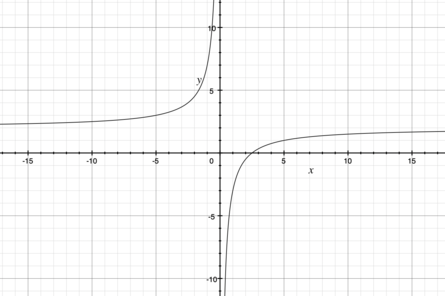# Sketch the graph of the following function. Indicate where the function is increasing or...

## Question:

Sketch the graph of the following function. Indicate where the function is increasing or decreasing, where any relative extrema occur, where asymptotes occur, where the graph is concave up or concave down, where any points of inflection occur, and where any intercepts occur. {eq}f(x) = \frac{2x - 5}{x} {/eq}

## Simple Analysis of Function

To find the extrema, intervals of increasing and decreasing, inflection points and intervals of concavity up and down, we need to find the function's first and second derivatives. Zeroes of the first one are extrema, intervals between them are intervals of increasing and decreasing(where the derivative is positive and negative respectively), zeroes of the second derivative are inflection points, intervals between them are intervals of concavity up and down(where the second derivative is positive and negative respectively).

Here is a graph of the function:Firstly, let's find its first derivative and its zeroes.

{eq}\displaystyle f'(x) = \frac{(2x-5)'\cdot...

Become a Study.com member to unlock this answer! Create your account